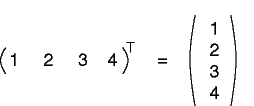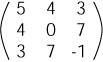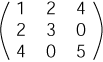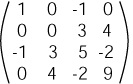The transpose of a row matrix is a column matrix. And the transpose of a column matrix is a row matrix.

# Symmetric

A symmetric matrix is equal to its transpose: AT = A.

A symmetric matrix must be a square matrix. Here are some symmetric matrices:In terms of its elements, if a matrix is symmetric, then aij = aji.

### QUESTION 16:

Is the zero matrix symmetric?